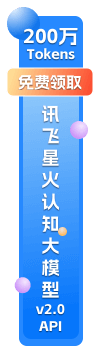API接口，开发服务，免费咨询服务

# Go 常见错误之一：值拷贝和 for 循环中的单一变量

``````package main

import (
"fmt"
"time"
)

type field struct {
name string
}

func (p *field) print() {
fmt.Println(p.name)
}

func main() {
data := []field{{"one"},{"two"},{"three"}}

for _,v := range data {
go v.print()
}

time.Sleep(3 * time.Second)
//goroutines print: three, three, three
}``````

``````package main

import (
"fmt"
"time"
)

type field struct {
name string
}

func (p *field) print() {
fmt.Println(p.name)
}

func main() {
data := []*field{{"one"},{"two"},{"three"}}

for _,v := range data {
v := v
go v.print()
}

time.Sleep(3 * time.Second)
//goroutines print: one, two, three
}``````

slice 是非指针

``````
data := []field{{"one"},{"two"},{"three"}}

for _,v := range data {
pp := (*field).print
go pp(&v) //非 pointer
}``````

slice 是指针

``````
data := []*field{{"one"},{"two"},{"three"}}

for _,v := range data {
pp := (*field).print
go pp(v) // pointer
}``````

``````
if rand.Intn(100) > 20 {
time.Sleep(1 * time.Second)
}``````API资讯

API接口，开发服务，免费咨询服务
Go 常见错误之一：值拷贝和 for 循环中的单一变量

``````package main

import (
"fmt"
"time"
)

type field struct {
name string
}

func (p *field) print() {
fmt.Println(p.name)
}

func main() {
data := []field{{"one"},{"two"},{"three"}}

for _,v := range data {
go v.print()
}

time.Sleep(3 * time.Second)
//goroutines print: three, three, three
}``````

``````package main

import (
"fmt"
"time"
)

type field struct {
name string
}

func (p *field) print() {
fmt.Println(p.name)
}

func main() {
data := []*field{{"one"},{"two"},{"three"}}

for _,v := range data {
v := v
go v.print()
}

time.Sleep(3 * time.Second)
//goroutines print: one, two, three
}``````

slice 是非指针

``````
data := []field{{"one"},{"two"},{"three"}}

for _,v := range data {
pp := (*field).print
go pp(&v) //非 pointer
}``````

slice 是指针

``````
data := []*field{{"one"},{"two"},{"three"}}

for _,v := range data {
pp := (*field).print
go pp(v) // pointer
}``````

``````
if rand.Intn(100) > 20 {
time.Sleep(1 * time.Second)
}``````• 短信API服务
• 银行卡四元素检测[简]
• 身份证实名认证
• 手机状态查询
• 三网手机实名制认证[简]
• 身份证OCR识别
• 证件识别
• 企业工商信息

• 短信API服务
• 银行卡四元素检测[简]
• 身份证实名认证
• 手机状态查询
• 三网手机实名制认证[简]
• 身份证OCR识别
• 证件识别
• 企业工商信息
• 确定×0512-88869195

Data Drives The Future